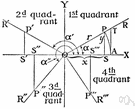# trigonometry

Also found in: Thesaurus, Acronyms, Encyclopedia, Wikipedia.

## trig·o·nom·e·try

(trĭg′ə-nŏm′ĭ-trē)
n.
The branch of mathematics that deals with the relationships between the sides and the angles of triangles and the calculations based on them, particularly the trigonometric functions.

[New Latin trigōnometria : Greek trigōnon, triangle; see trigon + Greek -metriā, -metry.]

## trigonometry

(ˌtrɪɡəˈnɒmɪtrɪ)
n
(Mathematics) the branch of mathematics concerned with the properties of trigonometric functions and their application to the determination of the angles and sides of triangles. Used in surveying, navigation, etc. Abbreviation: trig
[C17: from New Latin trigōnometria from Greek trigōnon triangle]

## trig•o•nom•e•try

(ˌtrɪg əˈnɒm ɪ tri)

n.
the branch of mathematics that deals with the relations between the sides and angles of plane or spherical triangles, and the calculations based on them.
[1605–15; < New Latin trigōnometria; see trigon, -o-, -metry]

## trig·o·nom·e·try

(trĭg′ə-nŏm′ĭ-trē)
The study of the properties and uses of trigonometric functions.

## trigonometry

the branch of mathematics that treats the measurement of and relationships between the sides and angles of plane triangles and the solid figures derived from them. — trigonometric, trigonometrical, adj.
ThesaurusAntonymsRelated WordsSynonymsLegend:
 Noun 1trigonometry - the mathematics of triangles and trigonometric functionstrigmath, mathematics, maths - a science (or group of related sciences) dealing with the logic of quantity and shape and arrangementpure mathematics - the branches of mathematics that study and develop the principles of mathematics for their own sake rather than for their immediate usefulnessspherical trigonometry - (mathematics) the trigonometry of spherical trianglestriangulation - a trigonometric method of determining the position of a fixed point from the angles to it from two fixed points a known distance apart; useful in navigation
Translations
trigonometrie
trigonometria
trigonometri

## trigonometry

[ˌtrɪgəˈnɒmɪtrɪ] N

## trigonometry

[ˌtrɪgəˈnɒmətri] n

## trigonometry

[ˌtrɪgəˈnɒmɪtrɪ] ntrigonometria
References in classic literature ?
This was something new and very interesting, and he felt that it was of greater import than trigonometry, which he never could understand.
I worked off a year's trigonometry that summer, and began Virgil alone.
In the alchemy of his brain, trigonometry and mathematics and the whole field of knowledge which they betokened were transmuted into so much landscape.
'"EDUCATION.--At Mr Wackford Squeers's Academy, Dotheboys Hall, at the delightful village of Dotheboys, near Greta Bridge in Yorkshire, Youth are boarded, clothed, booked, furnished with pocket-money, provided with all necessaries, instructed in all languages living and dead, mathematics, orthography, geometry, astronomy, trigonometry, the use of the globes, algebra, single stick (if required), writing, arithmetic, fortification, and every other branch of classical literature.
"'When my old tutor used to give me an exercise in trigonometry, it always took the shape of measuring heights.
He was the first to apply trigonometry, which he did not invent, to someone else's idea, using data he did not compile."
Table 12 illustrates that content of the unit 11"Introduction to Trigonometry" of National Curriculum 2006 for mathematics I-XIIis not exists in the grade VIII mathematics' textbook.
Research on the slab's rows and columns - inscribed with ancient cuneiform script - reveals that the Babylonians discovered trigonometry 1000 years before the Greeks.
Keywords: Trigonometry, Pythagorean Triples, Vedic mathematics.
He is well known for his contributions to spherical trigonometry , a wing of spherical geometry whose calculations are used most prevalently in astronomy, geodesy and navigation.
In another career, as a lecturer in mechanics at the university level, I have encountered a number of students whose basic geometry and trigonometry skills were woefully inadequate because they had been rushed through those courses in high school so that they could "move on" to calculus.

Site: Follow: Share:
Open / Close